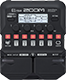# "Rat800" A Rat into an MS800

Discussion in 'Zoom G1/G1X Four' started by TPDLucas, Oct 25, 2020.

1. "Rat800" A Rat into an MS800Device: Zoom G1 Four
Firmware: 2.00

Name on device: Rat800
Optimized for: Phones/Speaker

Effects chain:This is a nice, rich, rat tone, even through a small practice amp,

Effect: "ZNR" (Dynamics), active - "yes"
"Detect" = GTRIN
"Depth" = 100
"Threshold" = 40
"Decay" = 0

Effect: "Squeak" (Overdrive / Distortion), active - "yes"
"Gain" = 75
"FLTR" = 45
"Volume" = 80
"DryMx" = 10

Effect: "Gt GEQ 7" (Filter), active - "yes"
"100" = 0.5
"200" = 1.0
"400" = 0.0
"800" = -9.0
"1.6k" = -1.0
"3.2k" = -1.5
"6.4k" = -2.0
"VOL" = 80

Effect: "MS 800" (Amp simulator), active - "yes"
"Input" = LO
"Bass" = 65
"Middle" = 45
"Treble" = 50
"Presence" = 30
"Gain" = 70
"Volume" = 85

Effect: "RCT4x12" (Cabinet), active - "yes"
"MIC" = OFF
"D57: D421" = 50
"Hi" = 60
"Lo" = 40

Patch Volume: 100